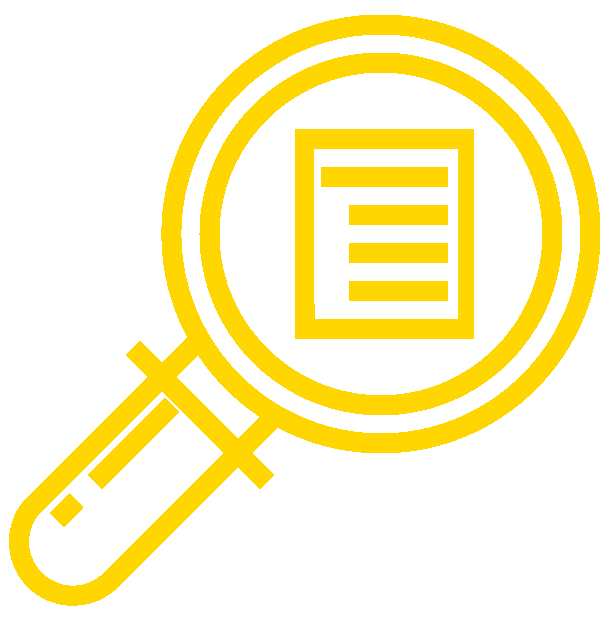## Learning OutcomesThe content, assignments, and assessments for this course are aligned to the following learning outcomes. A full list of course learning outcomes can be viewed here: Microeconomics Course Learning Outcomes.

## Module 1: Economic Thinking

• Explain what economics is and explain why it is important
• Use mathematics in common economic applications
• Use graphs in common economic applications

## Module 2: Choice in a World of Scarcity

• Explain the cost of choices and trade-offs
• Illustrate society’s trade-offs by using a production possibilities frontier, or curve
• Explain the assumption of rationality by individuals and firms

## Module 3: Supply and Demand

• Describe and differentiate between major economic systems
• Explain the determinants of demand
• Explain the determinants of supply
• Explain and graphically illustrate market equilibrium, surplus and shortage

## Module 4: Applications of Supply and Demand

• Analyze the economic effect of government setting price ceilings and floors
• Define, calculate, and illustrate consumer, producer, and total surplus
• Examine ways that supply and demand apply to labor and financial markets

## Module 5: Elasticity

• Explain the concept of elasticity
• Explain the price elasticity of demand and price elasticity of supply, and compute both using the midpoint method
• Explain and calculate other elasticities using common economic variables
• Explain the relationship between a firm’s price elasticity of demand and total revenue

## Module 6: Utility

• Describe the concept of utility and explain how consumers spend in order to maximize utility
• Explain how consumer utility changes when income or prices change
• Find consumer equilibrium using indifference curves and a budget constraint
• Describe the behavioral economics approach to understanding decision making

## Module 7: Production and Costs

• Explain production and the production function
• Calculate, graph and understand production costs in the short run
• Examine production choices in the long run

## Module 8: Perfect Competition

• Describe the characteristics of perfect competition and calculate costs, including fixed, variable, average, marginal, and total costs
• Analyze a firm’s profit margin
• Describe how perfectly competitive markets adjust to long run equilibrium

## Module 9: Monopoly

• Describe characteristics of monopolies
• Calculate and graph a monopoly’s costs, revenues, profit and losses
• Analyze strategies used to control monopolies

## Module 10: Monopolistically Competition and Oligopoly

• Describe the characteristics of a monopolistically competitive industry
• Calculate and graph a firm’s fixed, variable, average, marginal and total costs in monopolistic competition
• Describe characteristics of oligopolies

## Module 11: Public Goods

• Define and give examples of public goods and externalities
• Define and give examples of positive and negative externalities
• Analyze the efficacy of government policies to lessen negative externalities
• Analyze how the government promotes positive externalities

## Module 12: Labor Markets

• Analyze labor markets and how supply and demand interact to determine the market wage rate
• Explain how wages are determined when employers or employees hold labor market power
• Analyze the economic implications of discrimination and immigration policies

## Module 13: Income Distribution

• Explain poverty and the poverty trap
• Analyze and measure economic inequality

## Module 14: Globalization and Trade

• Define and calculate comparative advantage, and understand how countries choose which goods and services to trade internationally
• Explain how barriers to trade (like tariffs, quotas and non-tariff barriers) affect businesses, consumers and workers in the economy
• Differentiate between alternative international trade regimes and how they impact global trade

## Module 15: Exchange Rates and International Finance

• Define currency exchange rates and explain how they influence trade balances
• Analyze how supply and demand affects foreign currencies and exchange rates
• Explain how the balance of trade (surplus or deficit) affects the domestic economy

## Contribute!

Did you have an idea for improving this content? We’d love your input.